## Excel COUNTA function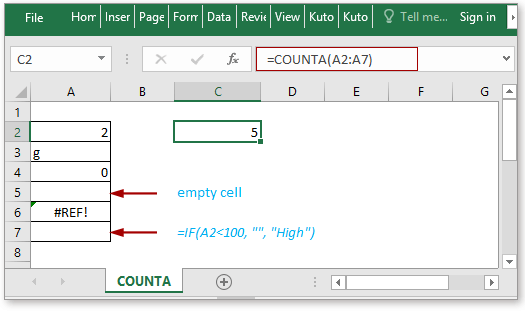### Description

The COUNTA function returns the number of cells that contain number, text, error value or the empty text “” (the result of a formula, for instance, A2=1, using =IF(A2<2,””,”FALSE”) returns empty text “”), but the COUNTA function does not count the number of empty cells.

### Syntax and arguments

Formula syntax

 COUNTA(value1,[value2], …)

Arguments

 Value1: Required, the first argument that you want to count, and it can be a cell, a value or a range. Value2: Optional，the argument that you want to count, and it can be a cell, a value or a range. There can be up to 255 arguments.

Return Value

The COUNTA function returns the number of cells excepted empty cells.

### Usage and Examples

Example1 Count cells in a range except empty cells

Supposing you want to count cells excluding empty cells, you can use below formula:

 =COUNTA(B2:E5)

Press Enter key to return the number of cells in the range B2:E5 except empty cells.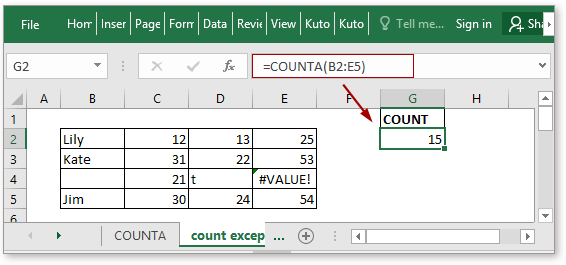Example2 Count cells in multiple ranges except empty cells

To count cell number of cells in multiple ranges, using formulas as below:

 =COUNTA(B18,C19,D19:D20,B20)

Or

 =COUNTA("KTE","KTE","KTO","KTW","OT")

Press Enter key to return the number of cells in the specified ranges.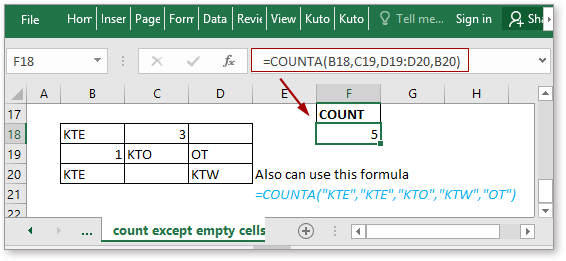Example3 Count cells including empty text “”

Sometimes, the cell looks empty but actually contains invisible characters or an empty text “” which is returned by formula. The COUNTA function count cells except the real empty cell.

Supposing there are some cells using the IF function as below screenshot shown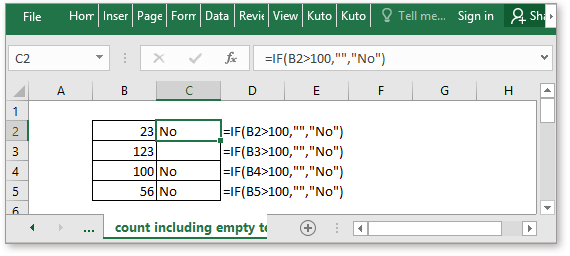Now use below formula to count the number of cells in the range B2:C5

 =COUNTA(B2:C5)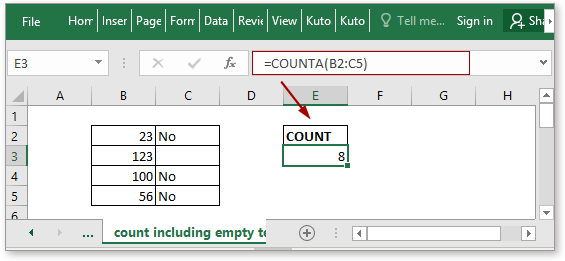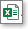Click to download COUNTA function sample file

#### Relative Functions:

• Excel COUNT Function
The COUNT function is used to count the number of cells that contain numbers, or count the numbers in a list of arguments. Take an instance, COUNT(1,2,3,4,11,2,1,0) returns 8.

• Excel COUNTBLANK Function
The COUNTBLANK function returns the number of empty cells. The COUNTBLANK function is a built-in statistical function, which can be used as a worksheet function (WS) in Excel.

• Excel COUNTIF Function
The COUNTIF function is a statistical function in Excel which is used to count the number of cells that meet a criterion.

• Excel COUNTIFS Function
The COUNTIFS function returns the number of cells that meet one single criterion or multiple criteria. The COUNNTIFS function is one of the built-in statistical function, and it can be used as a worksheet function (WS) in Excel.

#### Relative Articles:

• Count cells equal to
If you want to count cells exactly match a given criterion, you can choose the COUNTIF function or the SUMPRODUCT (combine with the EXACT function) function to count cells exactly equal to without case sensitive or with case sensitive.

• Count cells not equal to
To count the number of cells that contain values that not equal to a specified value, such as below screenshot shown. In Excel, you can use the COUNTIF function to quickly handle this job.

• Count cells equal to x or y
If you want to count cells as long as that meet at least one of given conditions (OR logic) in Excel, i.e. count cells equal to either x or y, you can use several COUNTIF formulas or combine the COUNTIF formula and SUM formula to handle this job.

• Count cells equal to x and y
Here in this tutorial, it will provide some formulas to talk about counting cells that match with all given conditions, which we call it the AND logic with the COUNTIFS function.

### The Best Office Productivity Tools

#### Kutools for Excel - Helps You To Stand Out From Crowd

 Popular Features: Find, Highlight or Identify Duplicates  |  Delete Blank Rows  |  Combine Columns or Cells without Losing Data  |  Round without Formula ... Super VLookup: Multiple Criteria  |  Multiple Value  |  Across Multi-Sheets  |  Fuzzy Lookup... Adv. Drop-down List: Easy Drop Down List  |  Dependent Drop Down List  |  Multi-select Drop Down List... Column Manager: Add a Specific Number of Columns  |  Move Columns  |  Toggle Visibility Status of Hidden Columns  |  Compare Columns to Select Same & Different Cells ... Featured Features: Grid Focus  |  Design View  |  Big Formula Bar  |  Workbook & Sheet Manager | Resource Library (Auto Text)  |  Date Picker  |  Combine Worksheets  |  Encrypt/Decrypt Cells  |  Send Emails by List  |  Super Filter  |  Special Filter (filter bold/italic/strikethrough...) ... Top 15 Toolsets:  12 Text Tools (Add Text, Remove Characters ...)  |  50+ Chart Types (Gantt Chart ...)  |  40+ Practical Formulas (Calculate age based on birthday ...)  |  19 Insertion Tools (Insert QR Code, Insert Picture from Path ...)  |  12 Conversion Tools (Numbers to Words, Currency Conversion ...)  |  7 Merge & Split Tools (Advanced Combine Rows, Split Excel Cells ...)  |  ... and more

Kutools for Excel Boasts Over 300 Features, Ensuring That What You Need is Just A Click Away...#### Office Tab - Enable Tabbed Reading and Editing in Microsoft Office (include Excel)

• One second to switch between dozens of open documents!
• Reduce hundreds of mouse clicks for you every day, say goodbye to mouse hand.
• Increases your productivity by 50% when viewing and editing multiple documents.
• Brings Efficient Tabs to Office (include Excel), Just Like Chrome, Edge and Firefox.